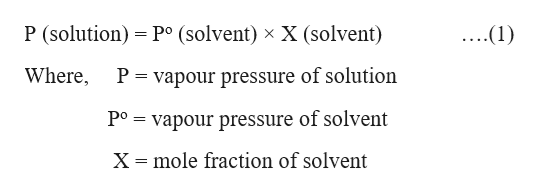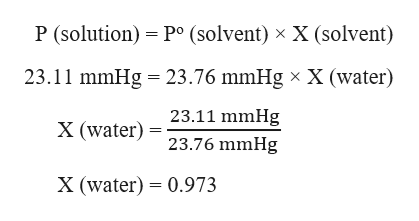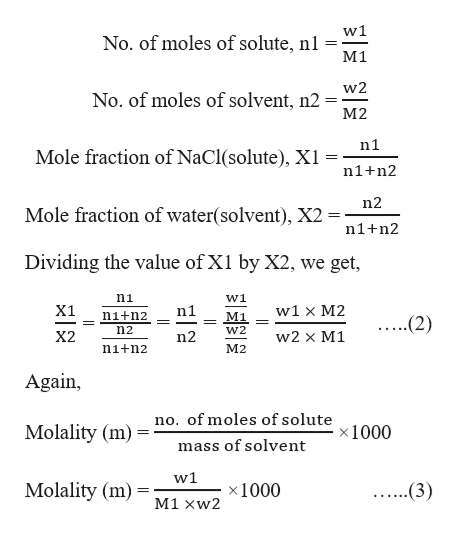# At 2°C, the vapor pressure of pure water is 23.76 mmHg and that of a certain seawater sample is 23.11 mmHg. Assuming that seawater contains only NaCl, estimate its molal concentration.

Question

At 2°C, the vapor pressure of pure water is 23.76 mmHg and that of a certain seawater sample is 23.11 mmHg. Assuming that seawater contains only NaCl, estimate its molal concentration.

check_circleExpert Solution
Step 1

Raoult’s law states that, at a certain temperature, the vapour pressure of a solution is equal to the vapour pressure of the pure solvent at the same temperature multiplied to the mole fraction of the solvent.help_outlineImage TranscriptioncloseP (solution) P (solvent) x X (solvent) ...(1) Where P = vapour pressure of solution vapour pressure of solvent Po mole fraction of solvent X fullscreen
Step 2

Given,

Vapor pressure of seawater sample (P) = 23.11 mmHg

Vapor pressure of pure water (Po) = 23.76 mmHg

Substituting these values in equation (1), we can calculate the mole fraction of water:help_outlineImage TranscriptioncloseP (solution) Po (solvent) x X (solvent) 23.11 mmHg 23.76 mmHg x X (water) 23.11 mmHg X (water) 23.76 mmHg X (water) 0.973 fullscreen
Step 3

The molality can be expressed in terms of mole fraction of solvent as follows:

Let, n1 = no. of moles of solute

n2 = no. of moles of solvent

w...help_outlineImage Transcriptionclosew1 No. of moles of solute, nl M 1 w2 No. of moles of solvent, n2 M2 n1 Mole fraction of NaCl(solute), Xi n1+n2 n2 Mole fraction of water(solvent), X2 n1+n2 Dividing the value of X1 by X2, we get, n1 w1 X1 n1 w1 x M2 n1+n2 M1 ...(2) n2 W2 X2 n2 w2 x M1 n1+n2 M2 Again no. of moles of solute Molality (m) x1000 mass of solvent w1 Molality (m) x1000 ..(3) M1 xw2 fullscreen

### Want to see the full answer?

See Solution

#### Want to see this answer and more?

Solutions are written by subject experts who are available 24/7. Questions are typically answered within 1 hour*

See Solution
*Response times may vary by subject and question
Tagged in

### Solutions